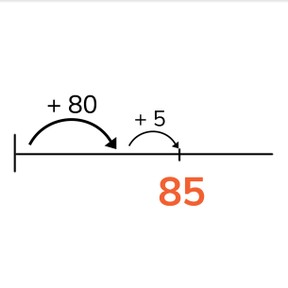Place value- numbers to 100 on the number line

# Place value- numbers to 100 on the number line

Place value- numbers to 100 on the number line8,000 schools use Gynzy92,000 teachers use Gynzy1,600,000 students use Gynzy

## General

Students learn to place numbers from 51 to 100 on the number line. They learn the tens and ones that make up numbers to 100 and can jump to these numbers on the number line.

1.NBT.B.2

## Relevance

Discuss with students that it is important to know how the numbers to 100 are put together so you can use them in calculation later.

## Introduction

The interactive whiteboard has a number of numbers and numbers written out in words. Drag the pairs together.

## Development

Show the tens and ones on the interactive whiteboard. Explain that tens are a group of 10 and ones are the numbers from 1 to 9. Show the MAB-blocks on the interactive whiteboard and/or show them in your classroom as well. Using the blocks, repeat the explanation of tens and ones. Show the TO-chart. Explain that the number 65 is made of 6 tens and 5 ones, and show how these jumps can be made on the number line. Point this out on the numbers next to the TO-chart. Show that you can also jump to 65 in a faster way by making jumps of 5 instead of jumps of 1. Practice as a class having students determine the tens and ones of a number. Discuss how to best jump to the number 46. Write the intermediate jumps on the interactive whiteboard. Next a few exercises are given in which students have to determine which number has been jumped to based on the given jumps on the number line.

Check that students know how to build numbers to 100 and place them on a number line by asking the following questions:
What are tens? How do you know?
What are ones? How do you know?

## Guided Practice

Students practice determining which number is jumped to on the number line.

## Closing

Discuss with students the importance of knowing how numbers to 100 are put together so you can do calculations with these numbers. Check that students understand what tens and ones are and that they can jump to a number on the number line. To practice this, set the students in pairs. The first student draws a number of jumps on a number line. The second student must say which number they drew. The students take turns drawing jumps and determining numbers.

## Teaching Tip

Students who have trouble can be supported by a deeper understanding of tens and ones. Have them use manipulatives or MAB-blocks to visually show the tens and ones.

## Instruction materials

MAB-blocks

### The online teaching platform for interactive whiteboards and displays in schools

• Save time building lessons

• Manage the classroom more efficiently

• Increase student engagement